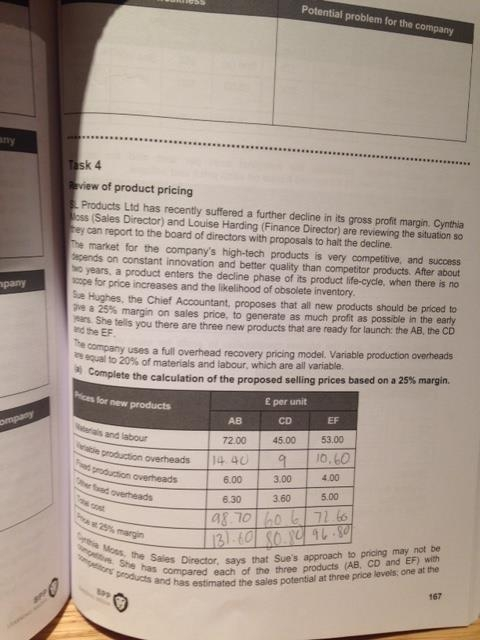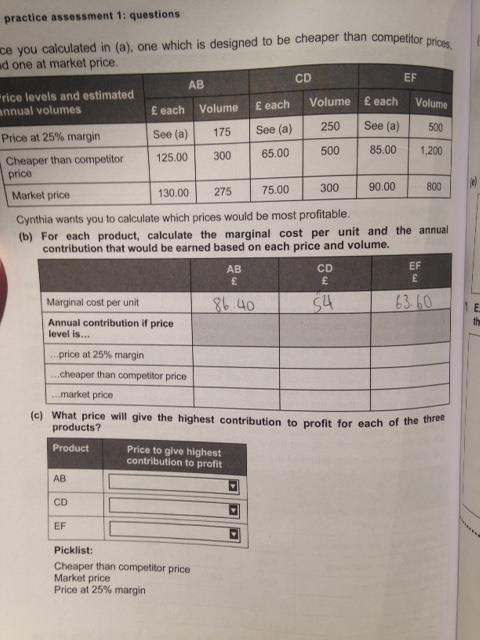# AAT Level 4 Synoptic -BPP practice assessment 1 task 4

Hi all,

Wondered if anyone could help me understand the workings of this question?

I have managed the first part, I am stuck on part b's workings.

Any help would be appreciated.

Thanks

•• Price @ 25% margin = 175*131.60=23030
175*86.40=15120
now the difference would be =7910
Cheaper than market price= 300*125=37500
300*86.40=25920
now the difference would be =11580
market price = 275*130=35750
275*86.40=23760
And the difference for this would be 11990

Now you can apply same method for CD and EF